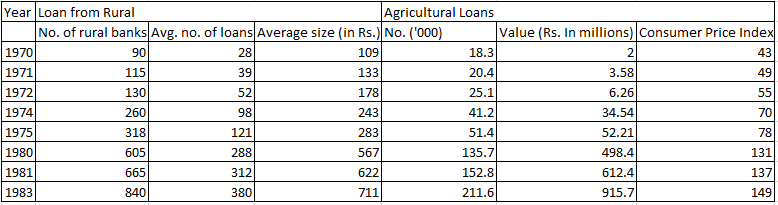### CAT 1997 Question Paper Question 29

Instructions

The table given below gives the annual details of loans from rural banks and agricultural loans over the years 1970 to 1983. Using this data answer the questions that follow.Question 29

# If the consumer price index for 1970 is to be taken as 105 and the indices for the subsequent years are to be corrected accordingly, by roughly how many points do the indices for 1983 and 1975 differ?

Solution

Difference between CPI of 1983 and 1975 = 149 - 78 = 71

If CPI of 1970, which is 43, is changed to 105, then the value of 71 changes to $$\frac{105}{43}*71$$ = 174

• All Quant CAT Formulas and shortcuts PDF
• 30+ CAT previous papers with solutions PDF